# MP5: LPC-10 Speech Synthesis¶

Speech is well modeled as an excitation (either white noise, impulse, or impulse train) filtered through a resonant filter. One of the more influential results of this model was the LPC-10 speech coder; modern cell phones are the result of lots of incremental improvements over the basic ideas that Atal put into LPC-10. In this MP, you'll create an LPC-10 speech synthesizer. The code package can be downloaded from http://courses.engr.illinois.edu/ece401/fa2020/ece401_20fall_mp5.zip.

In :
import numpy as np
import matplotlib.pyplot as plt
import matplotlib.figure
import wave,math
%matplotlib inline

In :
import importlib
import mp5

Out:
<module 'mp5' from '/Users/jhasegaw/Dropbox/mark/teaching/ece401/ece401labs/20fall/mp5/src/mp5.py'>

## Exploring the Data¶

This debugging page will analyze and resynthesize one waveform (if you want to resynthesize a different waveform, you can do it by changing the input filename below, or by running python mp5.py --waveform <myfile.wav>). The default waveform is data/file0.wav. Let's load it and look at it.

In :
with wave.open('data/file0.wav','rb') as w:
samplerate = w.getframerate()
nsamps = w.getnframes()
framelength = round(0.03*samplerate)
frameskip = round(0.015*samplerate)
nframes = 1+int(math.ceil((len(signal)-framelength)/frameskip))
order = 10

In :
t = np.linspace(0,nsamps/samplerate,nsamps)
fig=plt.figure(figsize=(14,4))
ax=fig.subplots()
ax.plot(t,signal)
print('Sample rate is %d'%(samplerate))

Sample rate is 11025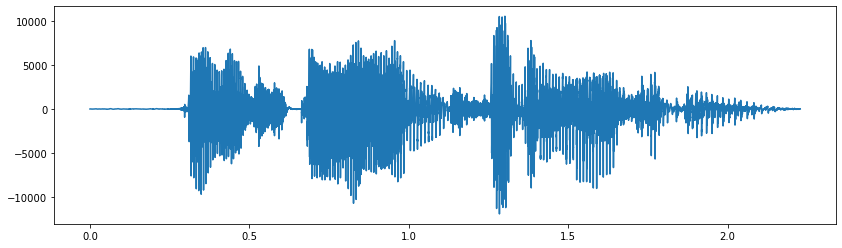In :
fig=plt.figure(figsize=(14,4))
ax=fig.subplots()
ax.plot(t[(t>0.3)&(t<0.5)],signal[(t>0.3)&(t<0.5)])

Out:
[<matplotlib.lines.Line2D at 0x7f8b4e4a4c40>]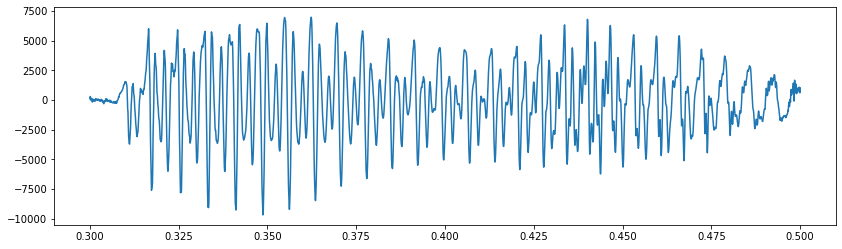## How to debug¶

Rather than printing every plot using separate blocks for both the distributed solution and your solution, instead, each of the following blocks will include lines to plot either one or the other. In order to switch between your solution and the distributed solution, you'll need to comment out the other one.

In :
import h5py
solutions = h5py.File('solutions.hdf5','r')


## LPC Analysis¶

First, let's analyze the signal, in order to find its LPC coefficients, pitch period, V/UV decision, and log RMS. This has five parts:

1. Chop the waveform into overlapping frames
2. Compute the autocorrelation coefficients in each frame
3. Compute the LPC coefficients in each frame
4. Find the pitch frequency in each frame
5. Find the level (energy, in decibels) of each frame

First, let's chop it into frames. We'll use a frame length of 30ms, and a frame skip of 15ms.

In :
importlib.reload(mp5)
framelength = round(0.03*samplerate)
frameskip = round(0.015*samplerate)
frames = solutions['frames'][:]
#frames = mp5.todo_frames(signal, framelength, frameskip)
time = np.linspace(0,framelength/samplerate,framelength,endpoint=False)
fig=plt.figure(figsize=(14,4))
axs=fig.subplots(3,2)
for row in range(3):
for col in range(2):
frame = int(row+3*col+20)
axs[row,col].plot(time,frames[frame,:])
axs[row,col].set_title('Frame %d (start: %gs)'%(frame,frame*0.015))
fig.tight_layout()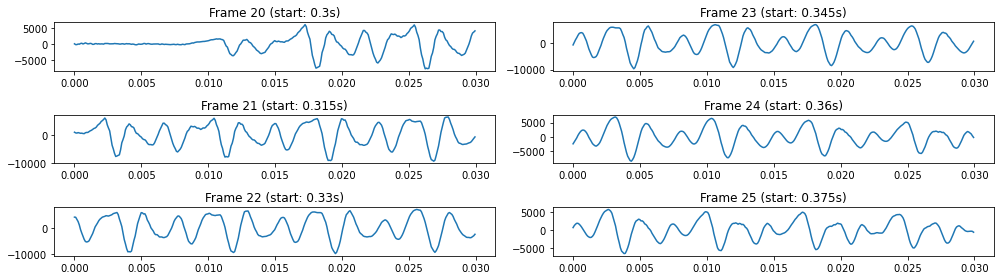Now we'll compute the autocorrelation coefficients.

In :
importlib.reload(mp5)
autocor = solutions['autocor'][:]
#autocor = mp5.todo_autocor(frames)
time = np.linspace(0,framelength/samplerate,framelength,endpoint=False)
fig=plt.figure(figsize=(14,4))
axs=fig.subplots(3,2)
for row in range(3):
for col in range(2):
frame = int(row+3*col+20)
axs[row,col].plot(time,autocor[frame,:])
axs[row,col].set_title('Frame %d (start: %gs)'%(frame,frame*0.015))
fig.tight_layout()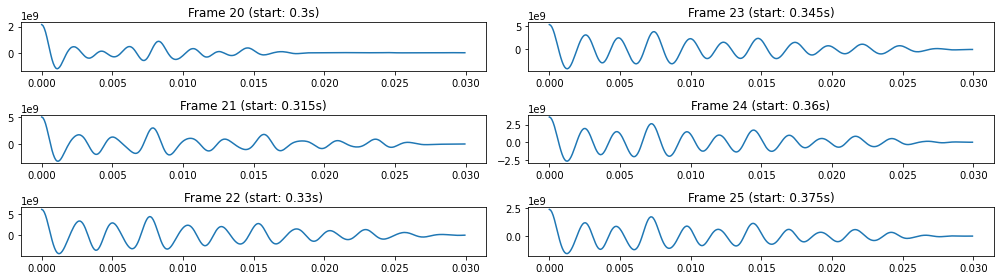As you can see, this syllable has a pitch period of about 8ms (F0=125Hz), and a first formant about 3X that frequency (about 375Hz). Let's find the LPC coefficients. First, we'll print all of the inputs and outputs (autocor, lpc, gamma, and Rmatrix) for frame 20, to make sure they make sense:

In :
importlib.reload(mp5)
lpc = solutions['lpc'][:]
gamma = solutions['gamma'][:]
Rmatrix = solutions['Rmatrix'][:]
#lpc, gamma, Rmatrix = mp5.todo_lpc(autocor, order)
print('\nAutocorrelation coefficients for frame 20:')
print(autocor[20,:4])
print('\ngamma vector for frame 20:')
print(gamma[20,:4])
print('\nR matrix for frame 20:')
print(Rmatrix[20,:4,:4])
print('\nLPC coefficients for frame 20:')
print(lpc[20,:])

Autocorrelation coefficients for frame 20:
[2.15571272e+09 2.07343650e+09 1.87198350e+09 1.57679194e+09]

gamma vector for frame 20:
[2.07343650e+09 1.87198350e+09 1.57679194e+09 1.19188747e+09]

R matrix for frame 20:
[[2.15571272e+09 2.07343650e+09 1.87198350e+09 1.57679194e+09]
[2.07343650e+09 2.15571272e+09 2.07343650e+09 1.87198350e+09]
[1.87198350e+09 2.07343650e+09 2.15571272e+09 2.07343650e+09]
[1.57679194e+09 1.87198350e+09 2.07343650e+09 2.15571272e+09]]

LPC coefficients for frame 20:
[ 1.43653741 -0.43496335  0.29996132 -0.29001224 -0.29092852  0.21309198
-0.00555242 -0.24599575  0.40135517 -0.16732984]


The LPC coefficients look pretty reasonable. Let's see if they correspond to a formant frequency at 375Hz, as expected. We can do that by plotting log|1/A(z)|, where z=exp(j omega).

In :
f = np.linspace(0,4000,100)
omega = 2*np.pi*f/samplerate
z = np.exp(1j*omega)
A = np.ones(f.shape, dtype=complex)
for k in range(order):
A -= lpc[23,k]*np.power(z,-(k+1))
fig=plt.figure(figsize=(14,4))
ax=fig.subplots()
ax.plot(f,20*np.log10(np.abs(1/A)))
ax.set_title('LPC synthesis filter for frame 20')

Out:
Text(0.5, 1.0, 'LPC synthesis filter for frame 20')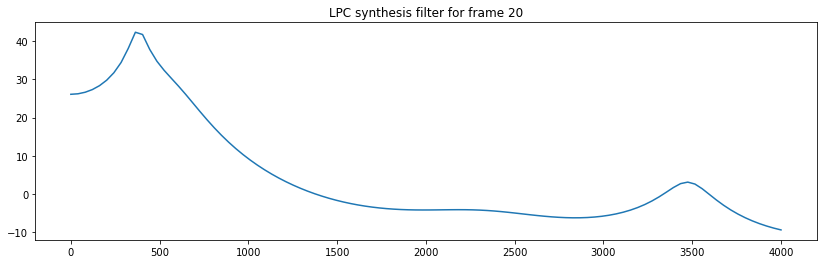Now let's try to extract the pitch and the level for each frame.

• "Level" is the average energy of each frame, expressed in decibels.
• Pitch is just the pitch period of each frame, in samples. If a frame is unvoiced (autocor[P]/autocor<0.25), the pitch should be set to 0. The function todo_framepitch should only consider pitch periods between 4ms and 13ms.
In :
importlib.reload(mp5)
framepitch = solutions['framepitch'][:]
framelevel = solutions['framelevel'][:]
#framepitch = mp5.todo_framepitch(autocor, samplerate)
#framelevel = mp5.todo_framelevel(frames)
fig=plt.figure(figsize=(14,8))
axs=fig.subplots(3,1)
axs.plot(signal)
axs.set_title('Waveform')
axs.plot(framepitch)
axs.set_title('Pitch period as a function of frame index')
axs.plot(framelevel)
axs.set_title('Level (energy in dB) as a function of frame index')
fig.tight_layout()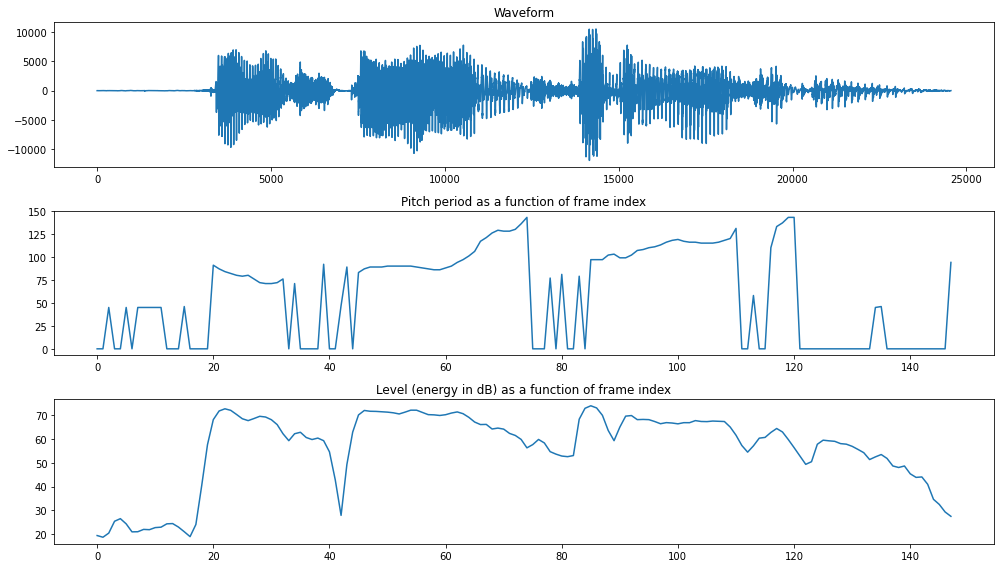## LPC Synthesis¶

Now let's resynthesize the speech, using the LPC-10 model! For this, we also have five parts:

1. Linearly interpolate between the levels of neighboring frames, in order to find a scaling factor for each waveform sample.
2. Linearly interpolate between the pitch periods of neighboring frames, in order to find the effective pitch period for each waveform sample.
3. Create the LPC-10 excitation signal, $e[n]$
4. Make sure the LPC coefficients are stable.
5. Generate the synthetic speech!
In :
importlib.reload(mp5)
samplelevel = solutions['samplelevel']
#samplelevel = mp5.todo_samplelevel(framelevel, frameskip)
fig=plt.figure(figsize=(14,6))
axs=fig.subplots(2,1)
axs.bar(np.arange(71,76),framelevel[71:76])
axs.set_title('Level of frames 71 through 76')
segment = samplelevel[71:76].reshape(-1)
axs.plot(71+np.arange(len(segment))/frameskip,segment)
axs.plot(np.arange(71,76),segment[::frameskip],'o')
axs.set_title('Level of the same frames, on a sample-by-sample basis')
fig.tight_layout()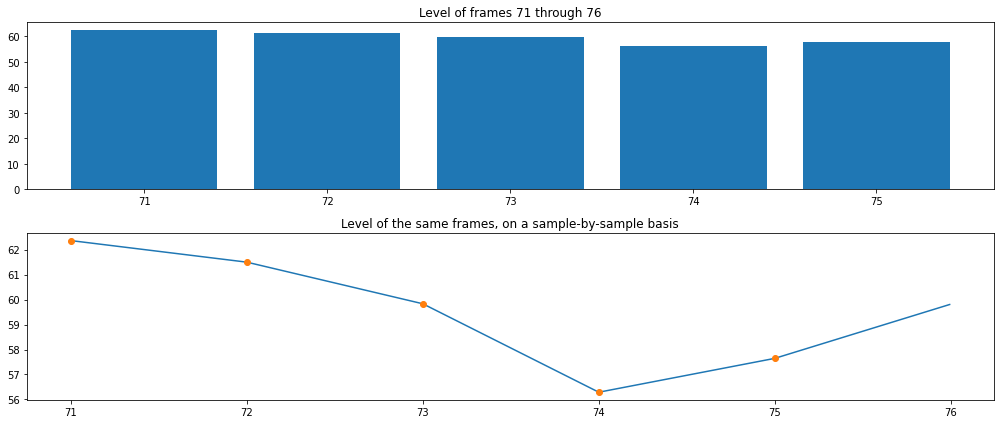In :
importlib.reload(mp5)
samplepitch = solutions['samplepitch'][:]
#samplepitch = mp5.todo_samplepitch(framepitch, frameskip)
fig=plt.figure(figsize=(14,4))
axs=fig.subplots(1,2)
axs.bar(np.arange(108,115),framepitch[108:115])
axs.set_title('Pitch of frames 108 through 115')
segment = samplepitch[108:115].reshape(-1)
axs.plot(108+np.arange(len(segment))/frameskip,segment)
axs.plot(np.arange(108,115),segment[::frameskip],'o')
axs.set_title('Pitch of the same frames, on a sample-by-sample basis')
fig.tight_layout()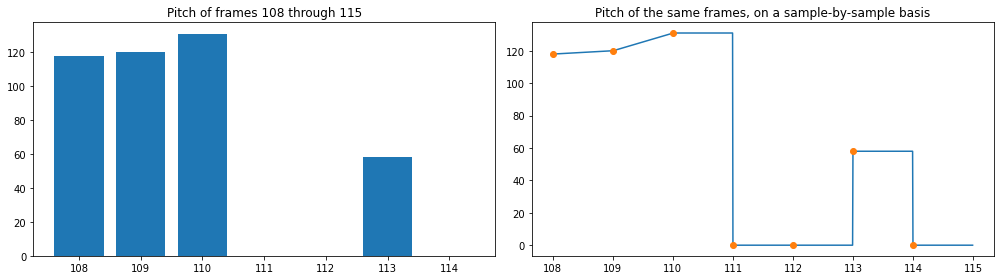In the above, notice how the pitch is linearly interpolated between frames, unless the next frame is unvoiced --- in that case, the pitch is set to be constant through the whole frame.

Now, let's generate the excitation signal. In voiced frames, we want an impulse train, with pulses spaced one per samplepitch samples, and with an amplitude of sqrt(samplepitch). In unvoiced frames, we want Gaussian pseudo-random noise.

In :
importlib.reload(mp5)
phase = solutions['phase'][:]
excitation = solutions['excitation'][:]
#phase,excitation = mp5.todo_excitation(samplepitch)
fig=plt.figure(figsize=(14,4))
ax=fig.subplots()
ax.plot(excitation[108:115].reshape(-1))
ax.set_title('Excitation signal for frames 108 through 115')

Out:
Text(0.5, 1.0, 'Excitation signal for frames 108 through 115')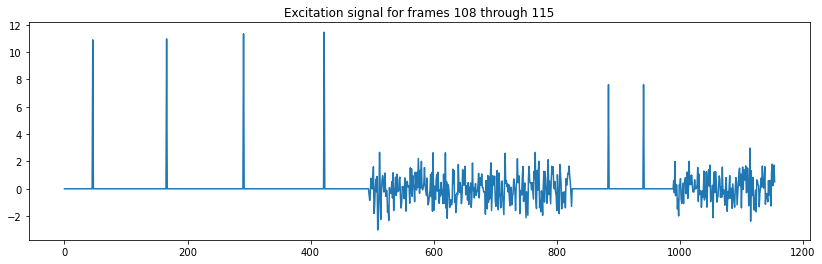In :
importlib.reload(mp5)
lpcpoly = solutions['lpcpoly'][:]
lpcroots = solutions['lpcroots'][:]
stableroots = solutions['stableroots'][:]
stablepoly = solutions['stablepoly'][:]
stablecofs = solutions['stablecofs'][:]
#lpcpoly,lpcroots,stableroots,stablepoly,stablecofs = mp5.todo_stable(lpc)
print('\nLPC coefficients in frame 23:')
print(lpc[23,:4])
print('\nLPC polynomial in frame 23:')
print(lpcpoly[23,:4])
print('\nLPC roots, frame 23:')
print(lpcroots[23,:4])
print('\nLPC roots after stabilization, frame 23:')
print(stableroots[23,:4])
print('\nLPC polynomial after stabilization, frame 23:')
print(stablepoly[23,:4])
print('\nLPC predictor coefficients after stabilization, frame 23:')
print(stablecofs[23,:4])

LPC coefficients in frame 23:
[ 1.80425001 -0.84416661  0.5529075  -0.85979625]

LPC polynomial in frame 23:
[ 1.         -1.80425001  0.84416661 -0.5529075 ]

LPC roots, frame 23:
[-0.7149209 +0.34118875j -0.7149209 -0.34118875j -0.37354376+0.85592004j
-0.37354376-0.85592004j]

LPC roots after stabilization, frame 23:
[ 1.         -1.80425001  0.84416661 -0.5529075 ]

LPC polynomial after stabilization, frame 23:
[-0.7149209 +0.34118875j -0.7149209 -0.34118875j -0.37354376+0.85592004j
-0.37354376-0.85592004j]

LPC predictor coefficients after stabilization, frame 23:
[ 1.80425001 -0.84416661  0.5529075  -0.85979625]


That wasn't very interesting.... in frame 23, the filter was already stable, so stabilizing it didn't do anything. Let's find a frame in which the original filter was unstable, and look at the difference.

In :
maxdiff = np.argmax(np.sum(np.abs(lpc-stablecofs),1))
print('\nLPC coefficients in frame %d:'%(maxdiff))
print(lpc[maxdiff,:4])
print('\nLPC polynomial in frame %d:'%(maxdiff))
print(lpcpoly[maxdiff,:4])
print('\nLPC roots, frame %d:'%(maxdiff))
print(lpcroots[maxdiff,:4])
print('\nLPC roots after stabilization, frame %d:'%(maxdiff))
print(stableroots[maxdiff,:4])
print('\nLPC polynomial after stabilization, frame %d:'%(maxdiff))
print(stablepoly[maxdiff,:4])
print('\nLPC predictor coefficients after stabilization, frame %d:'%(maxdiff))
print(stablecofs[maxdiff,:4])

LPC coefficients in frame 21:
[ 1.94979295 -1.45168493  1.40117461 -1.28710809]

LPC polynomial in frame 21:
[ 1.         -1.94979295  1.45168493 -1.40117461]

LPC roots, frame 21:
[-0.73224776+0.40691088j -0.73224776-0.40691088j -0.33661586+0.92332833j
-0.33661586-0.92332833j]

LPC roots after stabilization, frame 21:
[ 1.         -1.95169348  1.45122461 -1.39117057]

LPC polynomial after stabilization, frame 21:
[-0.73224776+0.40691088j -0.73224776-0.40691088j -0.3356656 +0.92072179j
-0.3356656 -0.92072179j]

LPC predictor coefficients after stabilization, frame 21:
[ 1.95169348 -1.45122461  1.39117057 -1.27557554]


OK, now let's synthesize the audio! Be sure to reshape the excitation into a signal before you run the LPC synthesis filter, so that the filter memory gets retained across frame boundaries.

In :
importlib.reload(mp5)
synthesis = solutions['synthesis'][:]
#synthesis = mp5.todo_synthesis(excitation,lpc,samplelevel)
fig=plt.figure(figsize=(14,6))
axs=fig.subplots(2,1)
axs.plot(signal)
axs.set_title('Original speech signal')
axs.plot(synthesis)
axs.set_title('Synthesized speech signal')
fig.tight_layout()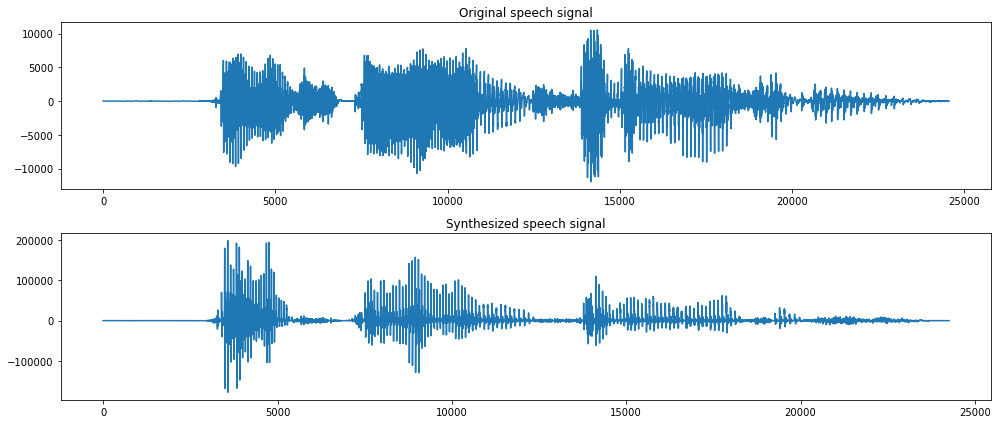## Conclusion¶

If you'd like to listen to the waveform, run python mp5.py, and then play result.wav.

In [ ]: